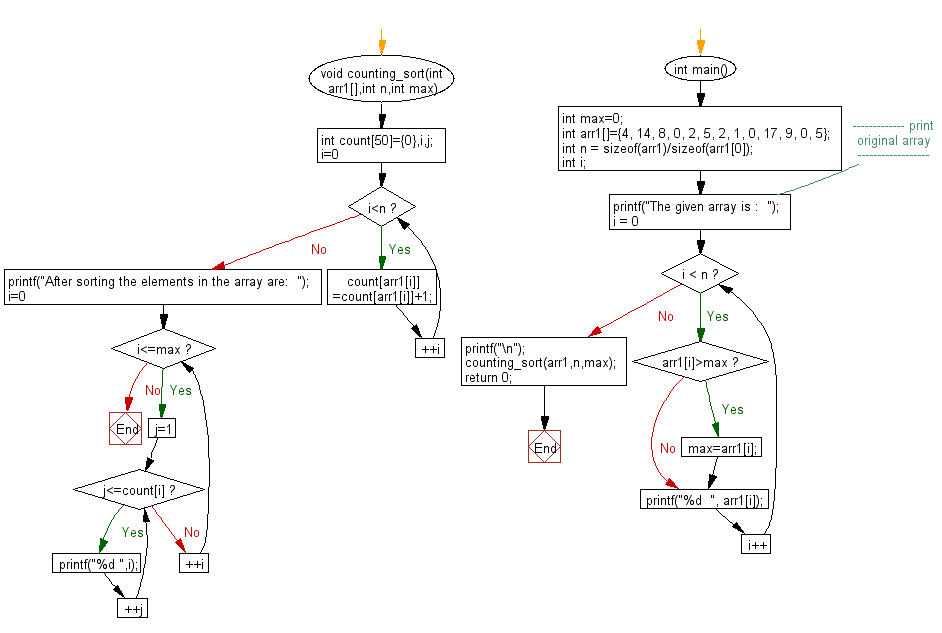﻿ C exercises: Return the counting sort on an array - w3resource# C Exercises: Return the counting sort on an array

## C Array: Exercise-59 with Solution

Write a program in C to return the counting sort on an array.

Sample Solution:

C Code:

``````#include <stdio.h>

void counting_sort(int arr1[],int n,int max)
{
int count={0},i,j;
for(i=0;i<n;++i)
count[arr1[i]]=count[arr1[i]]+1;
printf("After sorting the elements in the array are:  ");
for(i=0;i<=max;++i)
for(j=1;j<=count[i];++j)
printf("%d ",i);
}

int main()
{
int max=0;
int arr1[]={4, 14, 8, 0, 2, 5, 2, 1, 0, 17, 9, 0, 5};
int n = sizeof(arr1)/sizeof(arr1);
int i;
//------------- print original array ------------------
printf("The given array is :  ");
for(i = 0; i < n; i++)
{
if(arr1[i]>max)
max=arr1[i];
printf("%d  ", arr1[i]);
}
printf("\n");
//------------------------------------------------------
counting_sort(arr1,n,max);
return 0;
}
```
```

Sample Output:

```The given array is :  4  14  8  0  2  5  2  1  0  17  9  0  5
After sorting the elements in the array are:  0 0 0 1 2 2 4 5 5 8 9 14 17
```

Flowchart:C Programming Code Editor:

Improve this sample solution and post your code through Disqus.

What is the difficulty level of this exercise?

﻿